## ER Diagrams to Tables-

Before you go through this article, make sure that you have gone through the previous article on ER Diagrams to Tables.

After designing an ER Diagram,

• ER diagram is converted into the tables in relational model.
• This is because relational models can be easily implemented by RDBMS like MySQL , Oracle etc.
• The rules used for converting an ER diagram into the tables are already discussed.

In this article, we will discuss practice problems based on converting ER Diagrams to Tables.

## Problem-01:

Find the minimum number of tables required for the following ER diagram in relational model-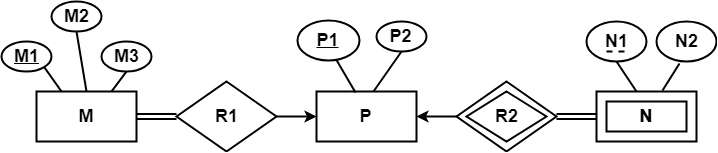## Solution-

Applying the rules, minimum 3 tables will be required-

• MR1 (M1 , M2 , M3 , P1)
• P (P1 , P2)
• NR2 (P1 , N1 , N2)

## Problem-02:

Find the minimum number of tables required to represent the given ER diagram in relational model-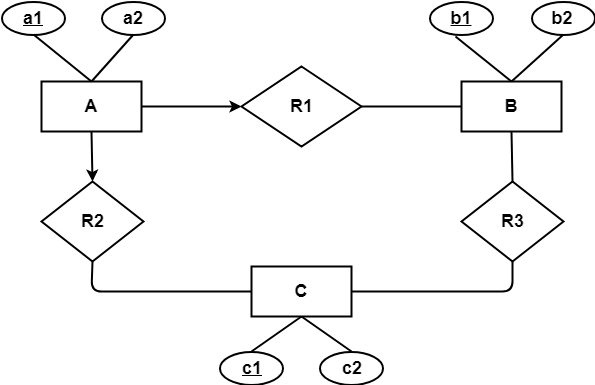## Solution-

Applying the rules, minimum 4 tables will be required-

• AR1R2 (a1 , a2 , b1 , c1)
• B (b1 , b2)
• C (c1 , c2)
• R3 (b1 , c1)

## Problem-03:

Find the minimum number of tables required to represent the given ER diagram in relational model-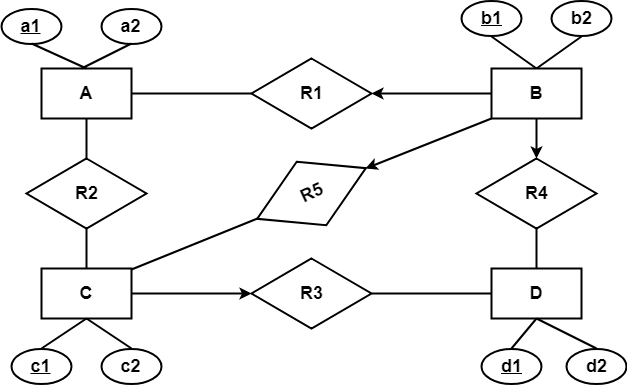## Solution-

Applying the rules, minimum 5 tables will be required-

• BR1R4R5 (b1 , b2 , a1 , c1 , d1)
• A (a1 , a2)
• R2 (a1 , c1)
• CR3 (c1 , c2 , d1)
• D (d1 , d2)

## Problem-04:

Find the minimum number of tables required to represent the given ER diagram in relational model-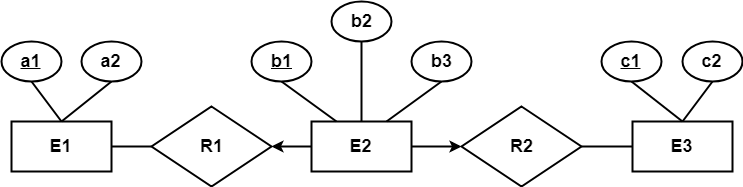## Solution-

Applying the rules, minimum 3 tables will be required-

• E1 (a1 , a2)
• E2R1R2 (b1 , b2 , a1 , c1 , b3)
• E3 (c1 , c2)

## Problem-05:

Find the minimum number of tables required to represent the given ER diagram in relational model-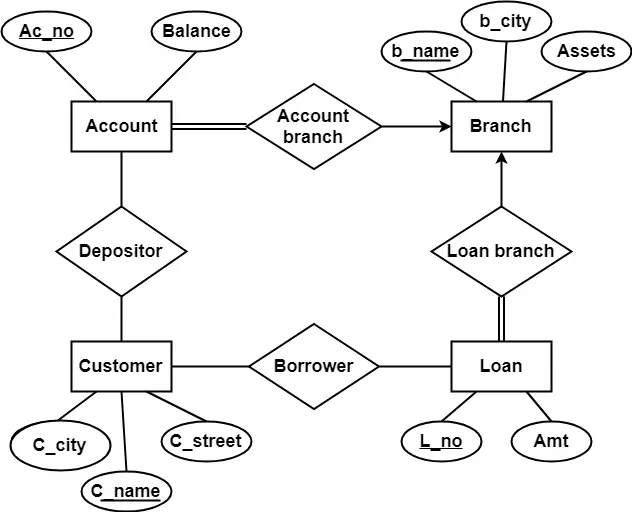## Solution-

Applying the rules that we have learnt, minimum 6 tables will be required-

• Account (Ac_no , Balance , b_name)
• Branch (b_name , b_city , Assets)
• Loan (L_no , Amt , b_name)
• Borrower (C_name , L_no)
• Customer (C_name , C_street , C_city)
• Depositor (C_name , Ac_no)

Next Article-Constraints in DBMS

Get more notes and other study material of Database Management System (DBMS).

Watch video lectures by visiting our YouTube channel LearnVidFun.

## Converting ER Diagrams to Tables-

After designing an ER Diagram,

• ER diagram is converted into the tables in relational model.
• This is because relational models can be easily implemented by RDBMS like MySQL , Oracle etc.

Following rules are used for converting an ER diagram into the tables-

## Rule-01: For Strong Entity Set With Only Simple Attributes-

A strong entity set with only simple attributes will require only one table in relational model.

• Attributes of the table will be the attributes of the entity set.
• The primary key of the table will be the key attribute of the entity set.

### Example-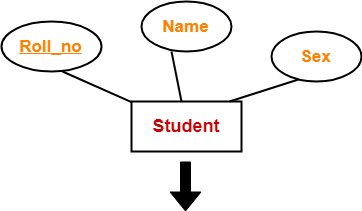Roll_no Name Sex

Schema : Student ( Roll_no , Name , Sex )

Also Read-Entity Sets in DBMS

## Rule-02: For Strong Entity Set With Composite Attributes-

• A strong entity set with any number of composite attributes will require only one table in relational model.
• While conversion, simple attributes of the composite attributes are taken into account and not the composite attribute itself.

### Example-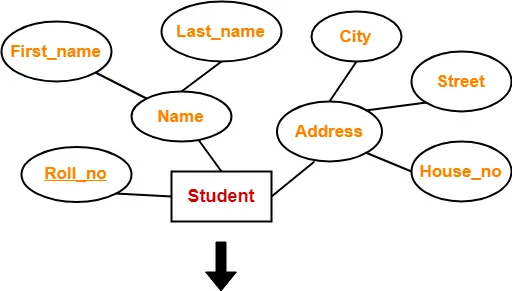Roll_no First_name Last_name House_no Street City

Schema : Student ( Roll_no , First_name , Last_name , House_no , Street , City )

Also Read-Types of Attributes in DBMS

## Rule-03: For Strong Entity Set With Multi Valued Attributes-

A strong entity set with any number of multi valued attributes will require two tables in relational model.

• One table will contain all the simple attributes with the primary key.
• Other table will contain the primary key and all the multi valued attributes.

### Example-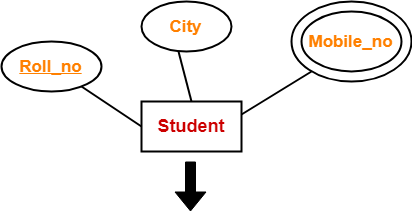Roll_no City

 Roll_no Mobile_no

## Rule-04: Translating Relationship Set into a Table-

A relationship set will require one table in the relational model.

Attributes of the table are-

• Primary key attributes of the participating entity sets
• Its own descriptive attributes if any.

Set of non-descriptive attributes will be the primary key.

### Example-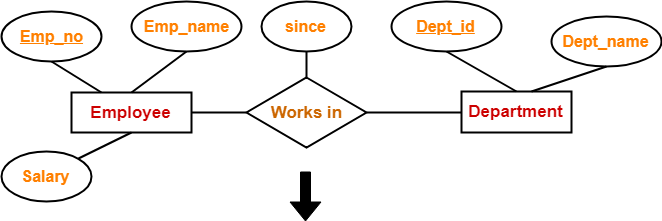Emp_no Dept_id since

Schema : Works in ( Emp_no , Dept_id , since )

## NOTE-

If we consider the overall ER diagram, three tables will be required in relational model-

• One table for the entity set “Employee”
• One table for the entity set “Department”
• One table for the relationship set “Works in”

## Rule-05: For Binary Relationships With Cardinality Ratios-

The following four cases are possible-

Case-01: Binary relationship with cardinality ratio m:n

Case-02: Binary relationship with cardinality ratio 1:n

Case-03: Binary relationship with cardinality ratio m:1

Case-04: Binary relationship with cardinality ratio 1:1

Also read-Cardinality Ratios in DBMS

### Case-01: For Binary Relationship With Cardinality Ratio m:n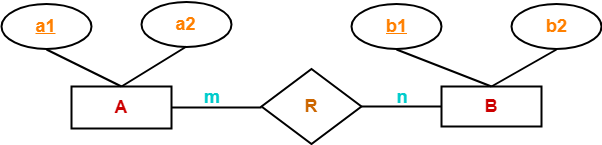Here, three tables will be required-

1. A ( a1 , a2 )
2. R ( a1 , b1 )
3. B ( b1 , b2 )

### Case-02: For Binary Relationship With Cardinality Ratio 1:n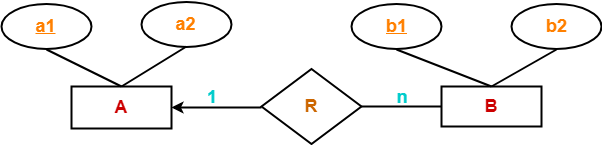Here, two tables will be required-

1. A ( a1 , a2 )
2. BR ( a1 , b1 , b2 )

NOTE- Here, combined table will be drawn for the entity set B and relationship set R.

### Case-03: For Binary Relationship With Cardinality Ratio m:1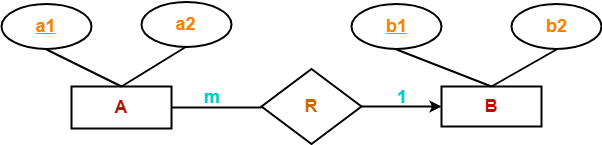Here, two tables will be required-

1. AR ( a1 , a2 , b1 )
2. B ( b1 , b2 )

NOTE- Here, combined table will be drawn for the entity set A and relationship set R.

### Case-04: For Binary Relationship With Cardinality Ratio 1:1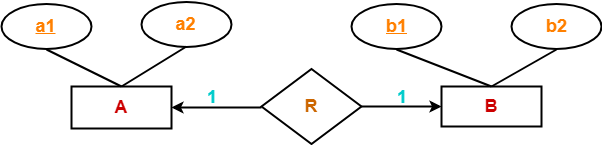Here, two tables will be required. Either combine ‘R’ with ‘A’ or ‘B’

Way-01:

1. AR ( a1 , a2 , b1 )
2. B ( b1 , b2 )

Way-02:

1. A ( a1 , a2 )
2. BR ( a1 , b1 , b2 )

### Thumb Rules to Remember

While determining the minimum number of tables required for binary relationships with given cardinality ratios, following thumb rules must be kept in mind-

• For binary relationship with cardinality ration m : n , separate and individual tables will be drawn for each entity set and relationship.
• For binary relationship with cardinality ratio either m : 1 or 1 : n , always remember “many side will consume the relationship” i.e. a combined table will be drawn for many side entity set and relationship set.
• For binary relationship with cardinality ratio 1 : 1 , two tables will be required. You can combine the relationship set with any one of the entity sets.

## Rule-06: For Binary Relationship With Both Cardinality Constraints and Participation Constraints-

• Cardinality constraints will be implemented as discussed in Rule-05.
• Because of the total participation constraint, foreign key acquires NOT NULL constraint i.e. now foreign key can not be null.

### Case-01: For Binary Relationship With Cardinality Constraint and Total Participation Constraint From One Side-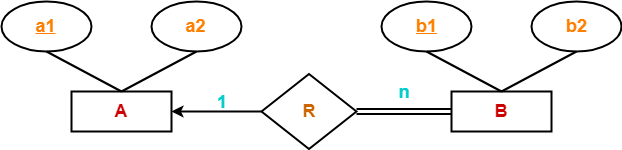Because cardinality ratio = 1 : n , so we will combine the entity set B and relationship set R.

Then, two tables will be required-

1. A ( a1 , a2 )
2. BR ( a1 , b1 , b2 )

Because of total participation, foreign key a1 has acquired NOT NULL constraint, so it can’t be null now.

### Case-02: For Binary Relationship With Cardinality Constraint and Total Participation Constraint From Both Sides-

If there is a key constraint from both the sides of an entity set with total participation, then that binary relationship is represented using only single table.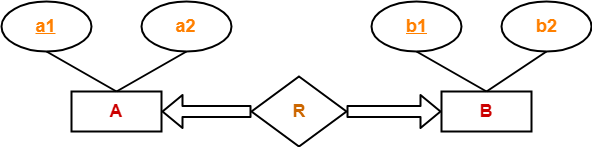Here, Only one table is required.

• ARB ( a1 , a2 , b1 , b2 )

## Rule-07: For Binary Relationship With Weak Entity Set-

Weak entity set always appears in association with identifying relationship with total participation constraint.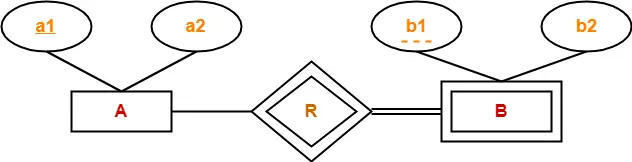Here, two tables will be required-

1. A ( a1 , a2 )
2. BR ( a1 , b1 , b2 )

Get more notes and other study material of Database Management System (DBMS).

Watch video lectures by visiting our YouTube channel LearnVidFun.